# Multiplication by Partitioning

Multiplication by Partitioning• In maths, partitioning means that we will split a number into smaller numbers, such as its tens and units.
• We can partition 14 into 10 + 4.
• 14 multiplied by 5 is the same as multiplying 10 and 4 by 5 separately and then adding the answers together.
• 10 multiplied by 5 is 50.
• 4 multiplied by 5 is 20.
• We add 40 and 20 to make 70.
• Therefore 14 multiplied by 5 is 70.
We partition our number into tens and units. We then multiply these values separately and add the values at the end.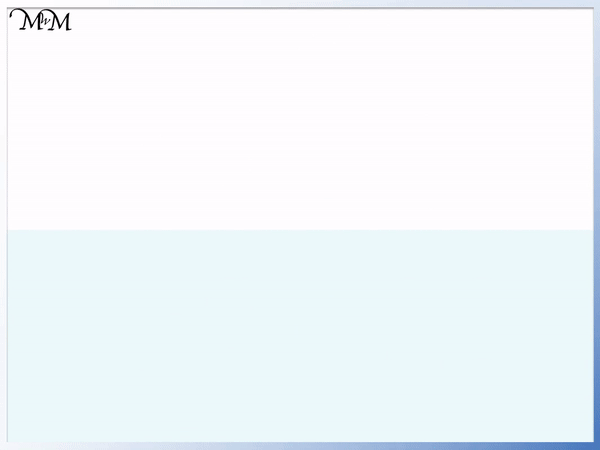• We have 27 multiplied by 6.
• We can partition 27 into 20 + 7.
• 20 x 6 = 120.
• 7 x 6 = 42.
• 120 + 42 = 162.
• Therefore 27 x 6 = 162.# What is the Multiplication by Partitioning Method?

The multiplication by partitioning method is a multiplication strategy that may be taught in primary schools to help work out larger multiplication calculations.

Multiplication by partitioning involves breaking a number into the values of its

, which we multiply separately and then add at the end.

In maths, partitioning means to break a larger number down into the sum of two or more numbers added together.

The most common way to partition numbers is to break them down into their hundreds, tens and units. We partition a number into the values of its individual digits.

This method is the most common multiplication strategy that we would teach a child to use to multiply a larger number

.

Here is an example of using the multiplication by partitioning method:To multiply 14 by 5, we could begin by partitioning 14. We partition our larger,

.The digits in ’14’ represent ‘1’ ten and ‘4’ units.

Therefore, 14 can be partitioned into 10 + 4.

Our method is to then multiply 5 by 10 and then multiply 5 by 4.

We multiply our partitioned parts separately.We begin by multiplying 10 by 5.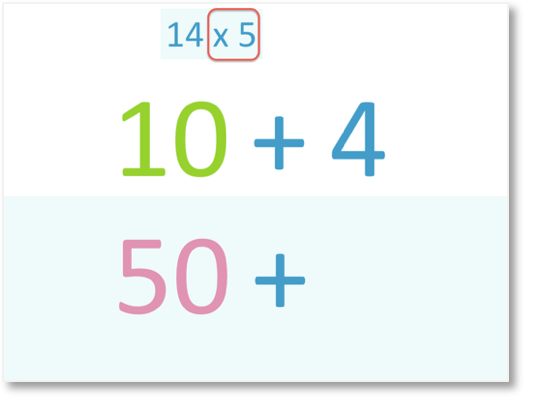5 x 10 = 50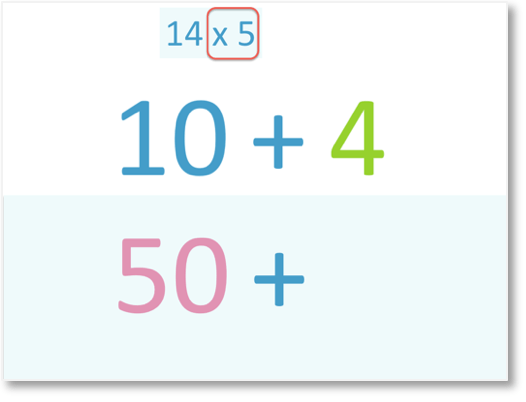Next, we multiply 5 by 4.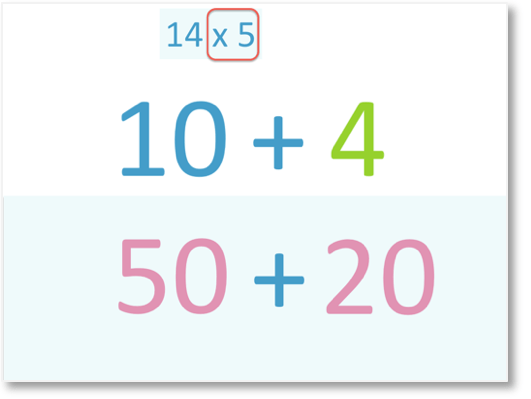5 x 4 = 20

Now that we have multiplied the parts separately, we must add the answers together.50 + 20 = 70

Therefore,

14 x 5 = 70.

The method of partitioning works because 10 lots of 5 plus 4 lots of 5 is the same as 14 lots of 5.

Here’s another example of using this method:To multiply 27 by 6, we can begin by partitioning 27.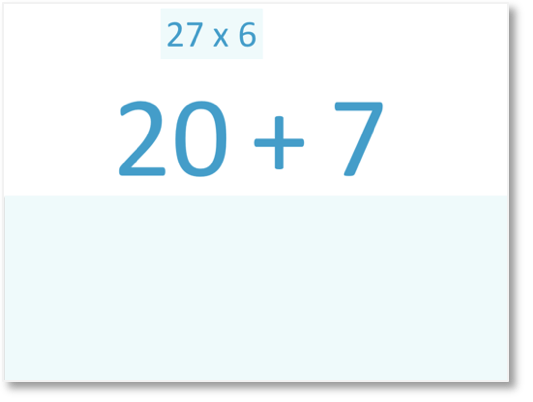27 can be partitioned into 20 and 7.

We want to find 27 lots of 6 and we know that we can make 27 lots of 6 by adding 20 lots of 6 to 7 lots of 6.

We will therefore multiply 6 by both 20 and 7 separately.

We will find 6 x 20 and then 6 x 7.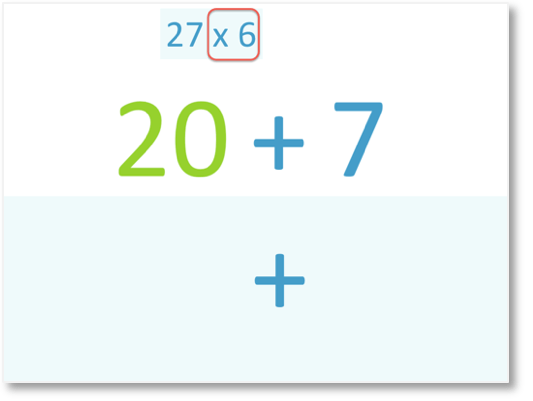We begin by multiplying 6 by 20.6 x 2 = 12

Therefore,

6 x 20 = 120

We can just multiply the digit ‘2’ by 6 and then times this by ten afterwards. When teaching this process, it is always easiest to multiply by the digit in the tens column using times tables and then multiply this by 10 afterwards.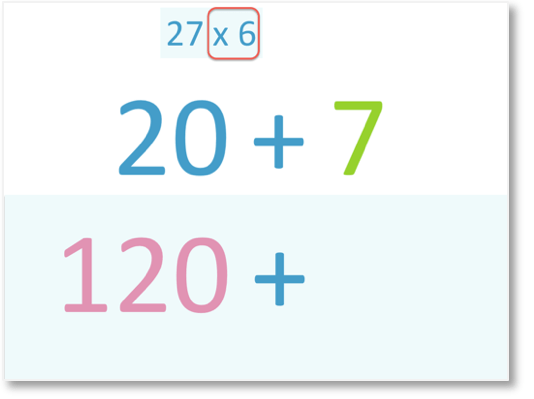Next, we multiply 6 by 7.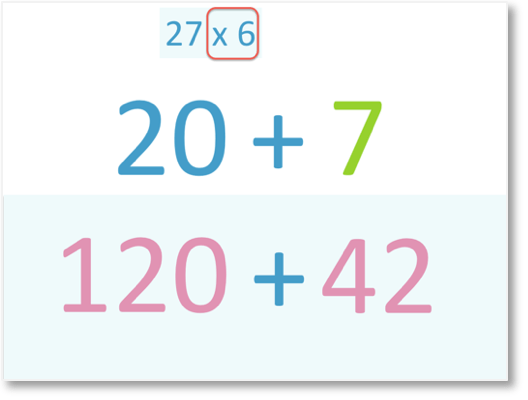6 x 7 = 42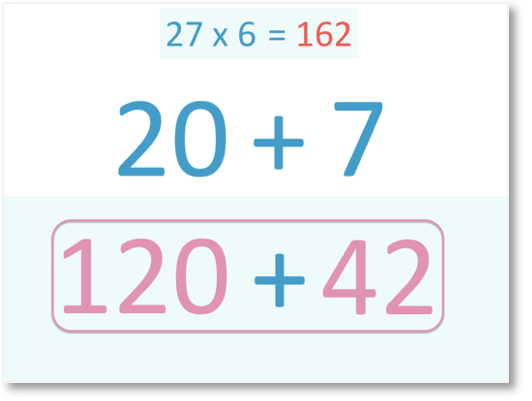Now that we have multiplied the parts separately, we must add the answers together.

120 + 42 = 162

Therefore,

27 x 6 = 162.

We found 27 lots of 6 by finding 20 lots of 6, 7 lots of 6 and then adding them together.

When teaching the multiplication by partitioning strategy, you should write down the result of the two multiplications and these values can be added with column addition.

However this method does lend itself to mental multiplication since there is unlikely to be much